# 英雄哪里出来

## 高斯消元

高斯消元，一般用于求解线性方程组AX = B（或 模线性方程组AX mod P = B），以四个未知数，四个方程为例，AX=B表示成4x4的矩        阵和4x1的矩阵相乘的形式：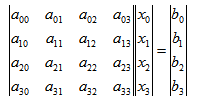对于n个方程，m个未知数的方程组，消元的具体步骤如下：

1、枚举第i (0 <= i < n) 行，初始化列为col = 0，每次从[i, n)行中找到第col列中元素绝对值最大的行和第i行进行交换(找到最大的行是为了在消元的时候把浮点数的误差降到最小)；

a) 如果第col列的元素全为0，放弃这一列的处理，col+1，i不变，转1)；

b) 否则，对于所有的行j (i < j < n)，如果a[j][col]不为0，则需要进行消元，以期第i行以下的第col列的所有元素都消为0（这一步就是线性代数中所说的初等行变换，具体的步骤就是将第j行的所有元素减去第i行的所有元素乘上一个系数，这个系数即a[j][col] / a[i][col]）。

2、重复步骤1) 直到n个方程枚举完毕或者列col == m。

3、判断解的情况：

a) 如果出现某一行，系数矩阵全为0，增广矩阵不全为0，则无解（即出现[0 0 0 0 0 b]，其中b不等于0的情况）；

b) 如果是严格上三角，则表明有唯一解；

c) 如果增广矩阵有k (k > 0)行全为0，那么表明有k个变量可以任意取值，这几个变量即自由变量；对于这种情况，一般解的范围是给定的，令解的取值有T个，自由变量有V个，那么解的个数就是 TV

O(n3)

ACM中的高斯消元题型一般涉及到的有：

1、浮点数消元

2、整数消元

3、模线性方程组

提供一个自己写的模线性方程的高斯消元模板：

1 #define MAXN 105
2 #define LL __int64
3
4 /*
5     高斯消元 - 同余方程
6         一般只要求求一个解/而且必然有解
7 */
8
9 LL GCD(LL a, LL b) {
10     if(!b) {
11         return a;
12     }
13     return GCD(b, a%b);
14 }
15
16 LL ExpGcd(LL a, LL b, LL &X, LL &Y) {
17      LL q, temp;
18      if( !b ) {
19          q = a; X = 1; Y = 0;
20          return q;
21      }else {
22         q = ExpGcd(b, a % b, X, Y);
23         temp = X;
24         X = Y;
25         Y = temp - (a / b) * Y;
26         return q;
27      }
28 }
29
30 LL Mod(LL a, LL b, LL c) {
31     if(!b) {
32         return 1 % c;
33     }
34     return Mod(a*a%c, b/2, c) * ( (b&1)?a:1 ) % c;
35 }
36
37 class GaussMatrix {
38 public:
39     int r, c;
40     LL d[MAXN][MAXN];
41     LL x[MAXN];        // 某个解集
42     LL  xcnt;          // 解集个数
43
44     LL abs(LL v) {
45         return v < 0 ? -v : v;
46     }
47
48     void swap_row(int ra, int rb) {
49         for(int i = 0; i <= c; i++) {
50             LL tmp = d[ra][i];
51             d[ra][i] = d[rb][i];
52             d[rb][i] = tmp;
53         }
54     }
55     void swap_col(int ca, int cb) {
56         for(int i = 0; i < r; i++) {
57             LL tmp = d[i][ca];
58             d[i][ca] = d[i][cb];
59             d[i][cb] = tmp;
60         }
61     }
62
63     void getAns(LL mod) {
64         for(int i = c-1; i >= 0; i--) {
65             LL tmp = d[i][c];
66             // d[i][i] * x[i] + (d[i][i+1]*x[i+1] +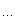+ d[i][c]*x[c]) = K*mod + tmp;
67             for(int j = i+1; j < c; j++) {
68                 tmp = ((tmp - d[i][j] * x[j]) % mod + mod) % mod;
69             }
70             // d[i][i] * x[i] = K * mod + tmp;
71             // d[i][i] * x[i] + (-K) * mod = tmp;
72             // a * x[i] + b * (-K) = tmp;
73             LL X, Y;
74             ExpGcd(d[i][i], mod, X, Y);
75             x[i] = ( (X % mod + mod) % mod ) * tmp % mod;
76         }
77     }
78
79     // -1 表示无解
80     LL gauss(LL mod) {
81         int i, j, k;
82         int col, maxrow;
83
84         // 枚举行，步进列
85         for(i = 0, col = 0; i < r && col < c; i++) {
86             //debug_print();
87             maxrow = i;
88             // 找到i到r-1行中col元素最大的那个值
89             for(j = i+1; j < r; j++) {
90                 if( abs(d[j][col]) > abs(d[maxrow][col]) ){
91                     maxrow = j;
92                 }
93             }
94             // 最大的行和第i行交换
95             if(maxrow != i) {
96                 swap_row(i, maxrow);
97             }
98             if( d[i][col] == 0 ) {
99                 // 最大的那一行的当前col值 等于0，继续找下一列
100                 col ++;
101                 i--;
102                 continue
103             }
104
105             for(j = i+1; j < r; j++) {
106                 if( d[j][col] ) {
107                     // 当前行的第col列如果不为0，则进行消元
108                     // 以期第i行以下的第col列的所有元素都消为0
109                     LL lastcoff = d[i][col];
110                     LL nowcoff = d[j][col];
111                     for(k = col; k <= c; k++) {
112                          d[j][k] = (d[j][k] * lastcoff - d[i][k] * nowcoff) % mod;
113                          if (d[j][k] < 0) d[j][k] += mod;
114                     }
115                 }
116             }
117             col ++;
118         }
119         // i表示从i往后的行的矩阵元素都为0
120         // 存在 (0 0 0 0 0 0 d[j][c]) (d[j][c] != 0) 的情况，方程无解
121         for(j = i; j < r; j++) {
122             if( d[j][c] ) {
123                 return -1;
124             }
125         }
126         // 自由变元数 为 (变量数 - 非零行的数目)
127         int free_num = c - i;
128
129         // 交换列，保证最后的矩阵为严格上三角，并且上三角以下的行都为0
130         for(i = 0; i < r && i < c; i++) {
131             if( !d[i][i] ) {
132                 // 对角线为0
133                 for(j = i+1; j < c; j++) {
134                     // 在该行向后找第一个不为0的元素所在的列，交换i和这一列
135                     if(d[i][j]) break;
136                 }
137                 if(j < c) {
138                     swap_col(i, j);
139                 }
140             }
141         }
142         xcnt = ( ((LL)1) << (LL)free_num );
143
144         getAns(mod);
145         return xcnt;
146     }
147
148     void debug_print() {
149         int i, j;
150         printf("-------------------------------\n");
151         for(i = 0; i < r; i++) {
152             for(j = 0; j <= c; j++) {
153                 printf("%d ", d[i][j]);
154             }
155             puts("");
156         }
157         printf("-------------------------------\n");
158     }
159 };
160

HDU 3359 Kind of a Blur

PKU 1830 开关问题

题意：给定N(N < 29)个开关，每个开关打开和关闭的时候会引起另外一个开关的变化，本来为打开的会变成关闭，本来关闭的会变成打开。给定N个开关的初始状态和终止状态，以及关联的开关关系，求共有多少种方案从初始状态变成终止状态（不计顺序，并且每个开关只能操作至多一次）。

题解：由于开关只有打开和关闭两种状态，所以对于每个开关的打开和关闭，组合一下总共有2^N种情况，枚举所有情况判可行性，对于这个数据量来说是不现实的，需要想办法优化。

( X * A[i]  +  X * A[i]  + ... +  X[n-1] * A[i][n-1]  ) % 2  =  (E[i] - S[i]);

1) 如果第j个开关的操作能够影响第i个开关的状态，那么A[i][j] = 1;

2) 如果第j个开关的操作不影响第i个开关的状态，那么A[i][j] = 0;

3) 特殊的A[i][i] = 1(开关本身的操作必然会影响自己的当前状态);

X[i]取值为0或1，这样就是N个N元一次方程组，利用高斯消元求解即可。将增广矩阵化简为上三角的形式后，剩余全为0的行的个数为自由变元的个数F（自由变元就是它在取值范围内可以取任意值，这题是个方阵，所以自由变元的个数等于全为0的行的个数），所以，由于开关一共两种状态，取值为0和1，所以总的解的个数为2^F。特殊的，如果某一行系数全为零，而增广矩阵最后一列对应行的值不为0，则表示无解。

HDU 3364 Lanterns

PKU 1830的简单变种。那题是用开关来控制开关，这题是用开关来控制灯，而且开关和灯的数目是不一样的，这样就导致了高斯矩阵并不是一个方阵，而是一个N*M的矩阵，

PKU 2065 SETI

(a1 * 10  +   a2 * 11  + ...  an * 1n) % P = C1

(a1 * 20  +   a2 * 21  + ...  an * 2n) % P = C2

(a1 * 30  +   a2 * 31  + ...  an * 3n) % P = C3

....

(a1 * n0  +   a2 * n1  + ...  an * nn) % P = Cn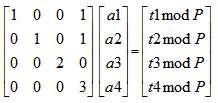PKU 3185 The Water Bowls

HDU 3571 N-dimensional Sphere

PKU 1288 Sly Number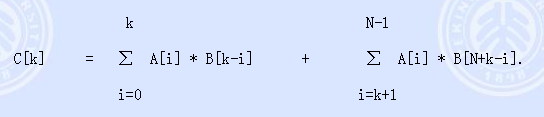1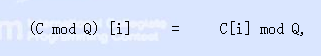2

C   =  (A*B)              +  ( A*B[N-1] + A*B[N-2] + ... + A[N-1]*B) ;

C   =  (A*B + A*B)  +  ( A*B[N-1] + ... + A[N-1]*B );

...

C[N-1] = (A*B[N-1] + A*B[N-2] +... A[N-1]*B);

SGU 173 Coins

1、从这一排硬币的Si(1 <= Si <= N, 1<= i <= M)位置取连续的K(2 <= K <= N)个硬币；

2、对这取出来的K个硬币进行一次循环左移操作；

3、扫描前K-1个硬币，如果第i个硬币字朝上(即为1)，并且Ai等于1，那么将第K个硬币进行一次翻转；

4、重复2) - 3) 操作Di(Di <= 106)次；

Ai求出来后，给定一个结束状态，模拟M*Di次逆向操作，每次操作需要进行字符串的右移(因为是逆向，所以要和题目的左移相反)操作，每次右移最多牵涉N次原子操作，所以总的复杂度为O(N*M*Di)，复杂度过大，但是注意到这里的N <= 50，所以我们可以将每个状态压缩到一个int64的整数中，A1A2A3..AK-1也可以压缩成一个int64的整数，右移操作可以通过位运算在O(1)的时间内解决。其中有一步会涉及到判断一个数的二进制表示中有多少个1的问题，网上各种面试题很多，不再累述，比较方便、效率也还可以的是采用树状数组中lowbit的思想，即利用 减去 (x&(-x)) (其中 x&(-x2^k kx二进制末尾0的个数），逐个将1消去，直到x = 0为止，迭代消去的次数就是1的个数

posted on 2014-06-08 19:55 英雄哪里出来 阅读(8046) 评论(2)  编辑 收藏 引用 所属分类: 算法专辑## #re: 高斯消元  回复更多评论

2014-11-18 18:20 | cdy

## #re: 高斯消元回复更多评论

2016-08-19 20:26 | fanyuheng

 只有注册用户登录后才能发表评论。 【推荐】超50万行VC++源码: 大型组态工控、电力仿真CAD与GIS源码库 相关文章: